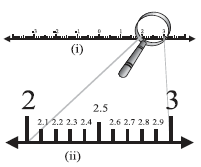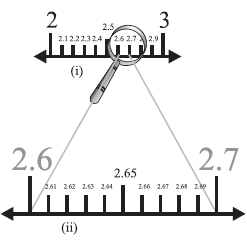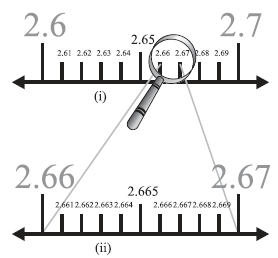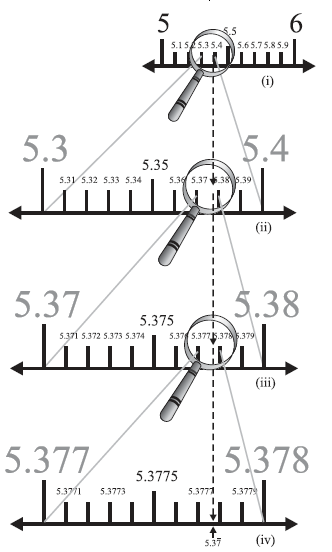Share

# Concept: Representing Real Numbers on the Number Line

#### notes

you have seen that any real number has a decimal expansion. This helps us to represent it on the number line. Let us see how.
Suppose we want to locate 2.665 on the number line. We know that this lies between 2 and 3.

So, let us look closely at the portion of the number line between 2 and 3. Suppose we divide this into 10 equal parts and mark each point of division as in Fig. 1.11 (i). Then the first mark to the right of 2 will represent 2.1, the second 2.2, and so on. You might be finding some difficulty in observing these points of division between 2 and 3 in Fig. 1.11 (i). To have a clear view of the same, you may take a magnifying glass and look at the portion between 2 and 3. It will look like what you see in Fig. 1.11 (ii). Now, 2.665 lies between 2.6 and 2.7. So, let us focus on the portion between 2.6 and 2.7 [See Fig. 1.12(i)]. We imagine to divide this again into ten equal parts. The first mark will represent 2.61, the next 2.62, and so on. To see this clearly, we magnify this as shown in Fig. 1.12 (ii).Fig. 1.11Fig. 1.12

Again, 2.665 lies between 2.66 and 2.67. So, let us focus on this portion of the number line [see Fig. 1.13(i)] and imagine to divide it again into ten equal parts. We magnify it to see it better, as in Fig. 1.13 (ii). The first mark represents 2.661, the next one represents 2.662, and so on. So, 2.665 is the 5th mark in these subdivisions.Fig. 1.13

We call this process of visualisation of representation of numbers on the number line, through a magnifying glass, as the process of successive magnification.
So, we have seen that it is possible by sufficient successive magnifications to visualise the position (or representation) of a real number with a terminating decimal expansion on the number line.

Let us now try and visualise the position (or representation) of a real number with a non-terminating recurring decimal expansion on the number line. We can look at appropriate intervals through a magnifying glass and by successive magnifications visualise the position of the number on the number line.

Example 11 : Visualize the representation of 5.bar37 on the number line upto 5 decimal places, that is, up to 5.37777.

Solution : Once again we proceed by successive magnification, and successively decrease the lengths of the portions of the number line in which 5.bar37 is located. First, we see that 5.bar37 is located between 5 and 6. In the next step, we locate 5.bar37 between 5.3 and 5.4. To get a more accurate visualization of the representation, we
divide this portion of the number line into 10 equal parts and use a magnifying glass to visualize that 5.bar37 lies between 5.37 and 5.38. To visualize 5.bar37 more accurately, we again divide the portion between 5.37 and 5.38 into ten equal parts and use a magnifying glass to visualize that 5.bar37 lies between 5.377 and 5.378. Now to visualize 5.bar37 still
more accurately, we divide the portion between 5.377 an 5.378 into 10 equal parts, and visualize the representation of 5.bar37 as in Fig. 1.14 (iv). Notice that 5.bar37 is located closer to 5.3778 than to 5.3777 [see Fig 1.14 (iv)].Fig. 1.14

Remark : We can proceed endlessly in this manner, successively viewing through a magnifying glass and simultaneously imagining the decrease in the length of the portion of the number line in which 5.37 is located. The size of the portion of the line we specify depends on the degree of accuracy we would like for the visualisation of the
position of the number on the number line.

You might have realised by now that the same procedure can be used to visualise a real number with a non-terminating non-recurring decimal expansion on the number line.

In the light of the discussions above and visualisations, we can again say that every real number is represented by a unique point on the number line. Further, every point on the number line represents one and only one real number.

### Shaalaa.com

Representing Real Numbers on the Number Line [00:15:55]
S
0%

S